## Producer Field Guide

HGD_Product
Producer Field Guide
HGD_Portfolio_Suite
Producer

Parameters Required for Orthorectification

SAR image orthorectification requires certain information about the sensor and the SAR image. Different sensors (TerraSAR-X, RADARSAT-2, and so forth) express these parameters in different ways and in different units. To simplify the design of our SAR tools and easily support future sensors, all SAR images and sensors are described using our SAR node model. The sensor-specific parameters are converted to a SAR node model on import or direct-read.

The following table lists the parameters of the SAR model and their units. These parameters can be viewed in SAR Model tab in SAR Model Properties (OrthoRadar) dialog.

SAR Parameters Required for Georeferencing

 Parameter Description Units sensor Sensor that produced the image orbit_direction Ascending (S->N) or descending (N->S) data_type Data type mode Original sensor acquisition mode coord_sys Coordinate system for ephemeris ('I' = Inertial, 'F' = Fixed Body or Earth rotating) year Year of image data collection month Month of image data collection day Day of image data collection doy Greenwich Mean Time (GMT) day of year of image data collection num_samples Number of samples in each line in the image (in range) num_lines Number of lines in the image (in azimuth) first_pt_secs_of_day Time of first ephemeris point (This parameter is updated during orbit adjustment) seconds of day first_pt_org Original time of first ephemeris point seconds of day time_interval Time interval between ephemeris points (This parameter is updated during orbit adjustment) seconds time_interval_org Original time interval between ephemeris points in seconds seconds image_start_time Image start time (azimuth direction) seconds of day image_end_time Image end time (azimuth direction) seconds of day image_duration Time duration of images (azimuth direction) seconds semimajor Semimajor spheroid axis of Earth model used during SAR processing meters semiminor Semiminor spheroid axis of Earth model used during SAR processing meters target_height Assumed height of scene above Earth model used during SAR processing meters look_side +90 = right-looking, -90 = left-looking degrees local_incidence_angle Incidence angle relative to a flat horizontal terrain at scene center (0 degrees = vertical reference, 90 degrees = horizontal reference) degrees wavelength Wavelength of sensor meters range_sampling_frequency Range sampling rate Hz azimuth_sampling_frequency Azimuth sampling rate Hz range_processing_bandwidth Range processing bandwidth Hz azimuth_processing_bandwidth Azimuth processing bandwidth Hz range_window_func Range window function range_window_coefficient Range window coefficient azimuth_window_func Azimuth window function azimuth_window_coefficient Azimuth window coefficient fdc_early_azimuth Doppler centroid in early azimuth fdc_late_azimuth Doppler centroid in late azimuth range_pix_spacing Slant or ground range pixel resolution meters azimuth_line_spacing Azimuth line resolution meters near_slant_range Slant range to near range pixel meters num_pos_pts Number of ephemeris points provided projection Ground or slant range projection (either PAR_GROUND or PAR_SLANT) gnd2slt_coeffs Coefficients used in polynomial transform from ground to slant range plane time_dir_pixels Time direction for increasing pixel (range) direction time_dir_lines Time direction for increasing azimuth (line) direction rsx Spacecraft X position (Earth Fixed Body coord system) in meters meters rsy Spacecraft Y position (Earth Fixed Body coord system) in meters meters rsz Spacecraft Z position (Earth Fixed Body coord system) meters vsx Spacecraft X velocity (Earth Fixed Body coord system) meters per second vsy Spacecraft Y velocity (Earth Fixed Body coord system) meters per second vsz Spacecraft Z velocity (Earth Fixed Body coord system) meters per second Calculated Ephemeris Coefficients orbit_state Status flag which indicates if the orbit has been adjusted rs_coeffs Coefficients used to model the sensor orbit positions as a function of time vs_coeffs Coefficients used to model the sensor orbit velocities as a function of time Subset Information Relative to the Original SAR Image sub_unity_subset Status flag indicating that the entire image is present sub_range_start Starting range sample of the current raster relative to the original image sub_range_end Ending range sample of the current raster relative to the original image sub_range_degrade Range sample compression factor of the current raster relative to the original image sub_range_num_samples Range number of samples of the current raster image sub_azimuth_start Starting azimuth line of the current raster relative to the original image sub_azimuth_end Ending azimuth line of the current raster relative to the original image sub_azimuth_degrade Azimuth line compression factor of the current raster relative to the original image sub_azimuth_num_lines Azimuth number of lines of the current raster image

Algorithm Description

Overview

The rectification process consists of several steps:

• ephemeris modeling and refinement (if GCPs are provided)
• sparse mapping grid generation
• output formation (including terrain corrections)

Each of these steps is described in detail in the following sections.

Ephemeris Coordinate System

The positions and velocities of the spacecraft are internally assumed to be in an Earth Fixed Body coordinate system. If the ephemeris are provided in inertial coordinate system, OrthoRadar converts them from inertial to Earth Fixed Body coordinates.

The Earth Fixed Body coordinate system is an Earth-centered Cartesian coordinate system that rotates with the Earth. The x-axis radiates from the center of the Earth through the 0 longitude point on the equator. The z-axis radiates from the center of the Earth through the geographic North Pole. The y-axis completes the right-handed Cartesian coordinate system.

Ephemeris Modeling

The platform ephemeris is described by three or more platform locations and velocities. To predict the platform position and velocity at some time (t):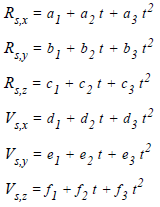Where:

Rs = sensor position

Vs = sensor velocity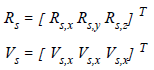To determine the model coefficients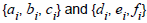, first do some preprocessing. Select the best three consecutive data points prior to fitting (if more than three points are available). The best three data points must span the entire image in time. If more than one set of three data points spans the image, then select the set of three that has a center time closest to the center time of the image.

Once a set of three consecutive data points is found, model the ephemeris with an exact solution.

Form matrix A: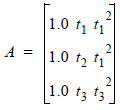Where t1, t2, and t3 are the times associated with each platform position.

Select t such that t = 0.0 corresponds to the time of the second position point.

Form vector b: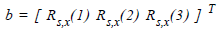Where Rs,x(i) is the x-coordinate of the i-th platform position (i =1: 3).

We wish to solve Ax = b where x is: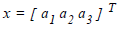To do so, use LU decomposition. The process is repeated for: Rs,y, Rs,z, Vs,x, Vs,y, and Vs,z

SAR Imaging Model

Before discussing the ephemeris adjustment, it is important to understand how to get from a pixel in the SAR image (as specified by a range line and range pixel) to a target position on the Earth [specified in Earth Centered System (ECS) coordinates or x, y, z]. This process is used throughout the ephemeris adjustment and the rectification itself.

For each range line and range pixel in the SAR image, the corresponding target location (Rt) is determined. The target location can be described as (lat, lon, elev) or (x, y, z) in ECS. The target can either lie on a smooth Earth ellipsoid or on a smooth Earth ellipsoid plus an elevation model.

In either case, the location of Rt is determined by finding the intersection of the Doppler cone, range sphere, and Earth model. In order to do this, first find the Doppler centroid and slant range for a given SAR image pixel.

Let i = range pixel and j = range line.

Time

Time T(j) is thus: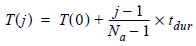Where:

T(0) = image start time

Na = number of range lines

tdur = image duration time

Doppler Centroid

The computation of the Doppler centroid fd to use with the SAR imaging model depends on how the data was processed. If the data was deskewed, this value is always 0. If the data is skewed, then this value may be a nonzero constant or may vary with i.

Slant Range

The computation of the slant range to the pixel i depends on the projection of the image. If the data is in a slant range projection, then the computation of slant range is straightforward: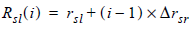Where:

Rsl(i) = slant range to pixel i

rsl = near slant range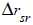= slant range pixel spacing

If the projection is a ground range projection, then this computation is potentially more complicated and depends on how the data was originally projected into a ground range projection by SAR processor.

Intersection of Doppler Cone, Range Sphere, and Earth Model

To find the location of the target Rt corresponding to a given range pixel and range line, the intersection of the Doppler cone, range sphere, and Earth model must be found. For an ellipsoid, these may be described as follows: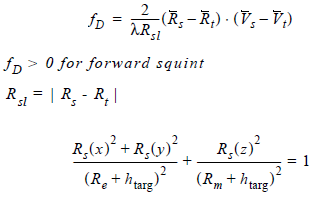Where:

Rs and Vs = platform position and velocity respectively

Vt = target velocity ( = 0, in this coordinate system)

Re = Earth semimajor axis

Rm = Earth semiminor axis

The platform position and velocity vectors Rs and Vs can be found as a function of time T(j) using the ephemeris equations developed previously.

The following figure graphically illustrates the solution for the target location given the sensor ephemeris, doppler cone, range sphere, and flat Earth model:

Doppler Cone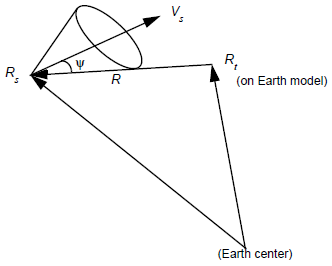There are three possible adjustments that can be made: along track, cross track, and radial. In OrthoRadar, the along track adjustment is performed separately. The cross track and radial adjustments are made simultaneously. These adjustments are made using residuals associated with GCPs. Each GCP has a map coordinate (such as lat, lon) and an elevation. Also, an SAR image range line and range pixel must be given. The SAR image range line and range pixel are converted to Rt using the method described previously (substituting htarg = elevation of GCP above ellipsoid used in SAR processing).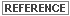For more information, see SAR Geocoding: Data and Systems Gunter, Schreier, Ed.

Orthorectification

The ultimate goal in orthorectification is to determine, for a given target location on the ground, the associated range line and range pixel from the input SAR image, including the effects of terrain.

To do this, there are several steps. First, take the target location and locate the associated range line and range pixel from the input SAR image assuming smooth terrain. This places you in approximately the correct range line. Next, look up the elevation at the target from the input DEM. The elevation, in combination with the known slant range to the target, is used to determine the correct range pixel. The data can now be interpolated from the input SAR image.

Sparse Mapping Grid

Select a block size M. For every Mth range line and Mth range pixel, compute Rt on a smooth ellipsoid (using the SAR Earth model), and save these values in an array. Smaller M implies less distortion between grid points.

Regardless of M and the total number of samples and lines in the input SAR image, always compute Rt at the end of every line and for the very last line. The spacing between points in the sparse mapping grid is regular except at the far edges of the grid.

Output Formation

For each point in the output grid, there is an associated Rt. This target should fall on the surface of the Earth model used for SAR processing, thus a conversion is made between the Earth model used for the output grid and the Earth model used during SAR processing.

The process of orthorectification starts with a location on the ground. The line and pixel location of the pixel to this map location can be determined from the map location and the sparse mapping grid. The value at this pixel location is then assigned to the map location. The following figure illustrates this process.

Sparse Mapping and Output Grids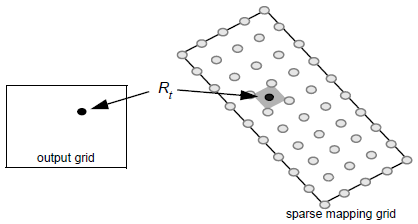Conversion to Real / Imaginary Data

The formula used for converting a block of complex 1-layer data into a 2-layer IQ (real/imaginary) output datastack, or converting a block of 2-layer input data into a 2-layer IQ (real/imaginary) output datastack is shown here. The conversion is available in Radar Conversions dialog. The magnitude and phase data is represented in the figure below.

real = magnitude * cos(phase)

imaginary = magnitude * sin(phase)

The above mathematical notation is 100% equivalent, simply expressed in a different form.

For radar systems, a complex number implies that the representation of a signal, or data file, needs measures of both magnitude and phase. In the context of digital SAR, a complex number can be also be represented by the real in-phase component (I) and the imaginary quadrature component (Q). The role of complex numbers is an essential part of the signal as signal phase is used to obtain high resolution.

• The set {magnitude, phase}, or {r,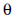} represents coordinates in polar form.
• The set {real, imaginary}, or { I, Q } represents coordinates in Cartesian form.

Magnitude and Phase Data as shown in the complex plane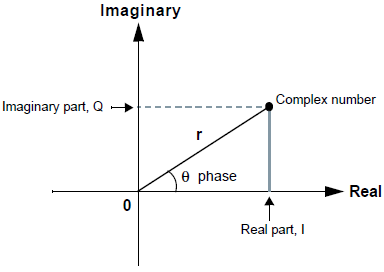Where:

Real = Real axis

Imaginary = Imaginary axis

r = Magnitude is defined as a dimensionless number describing the length of the backscatter vector in the complex domain.

phase = Relative interferometric phase angle in radians in the range 0 to 2*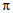, measured from the positive Real axis towards the backscatter vector.